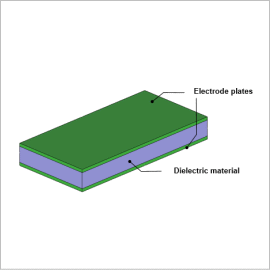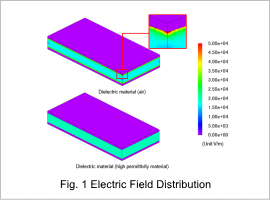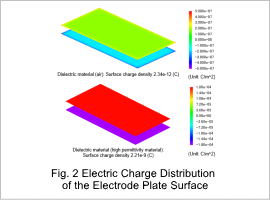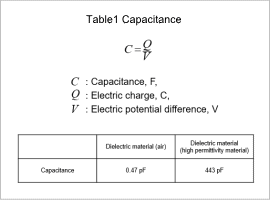## [JAC073] Analysis of the Capacitance of a Parallel Plate Capacitor

Remember me

*It is different from the service for JMAG WEB MEMBER (free member). Please be careful.
About authentication ID for JMAG website

### OverviewCapacitors are widely used as basic passive components in a variety of electric circuits. Parallel plate capacitors are taken up as the subject matter for how to obtain capacitance, a basic electric property, because they follow simple phenomena. Two electrode plates are placed in the air to face each other. When a constant electric potential difference is applied to them, they store an electric charge. The capacity of the charge stored in the electrode plates changes depending on the permittivity of the dielectric material between them.
This Application Note explains how to use JMAG to calculate the capacitance when the dielectric material is air and when it is a high permittivity material, while obtaining the amount of charge in each.

### Electric Field DistributionThe magnetic field distributions generated between the two electrode plates when they are in parallel and an electric potential difference is applied to them are shown in fig. 1. When the dielectric material is air, there is a large magnetic field that extends from the opposing faces of the electrode plates to the air, so there is a large magnetic field generated locally at the corner of the electrode.

### Capacitance Calculation

The electric charge distribution generated on the surfaces of the electrode plates is shown in fig. 2. When the high permittivity material is used, the amount of surface charge density is larger than that of air. The capacitance is calculated with the following formula by using the amount of surface charge density and the electric potential difference applied in the analysis. The result is shown in table 1.